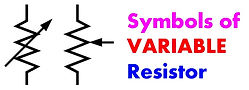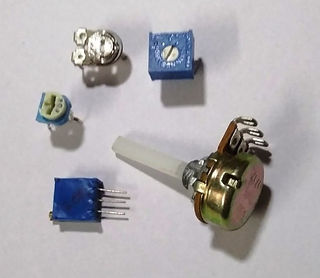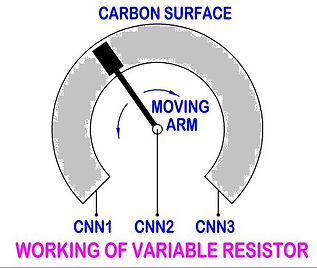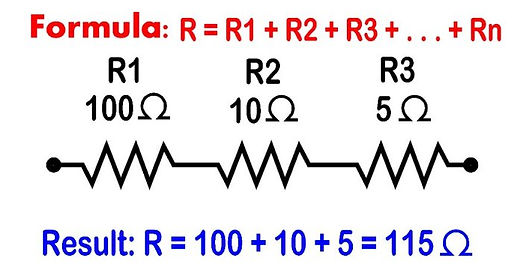top of page

## Resistors:

As the name indicates, Resistor reduces flow of current in a circuit. Resistor is highly used element in electronic circuit. The value of a resistor is called Resistance and its units are (i.e. measured in) Ohms. The symbol for Ohms is omega ( Ω ). The property of the material which exerts resistance is called resistivity and its units are rho ( ρ ).## Fixed Value Resistors:

The value of resistance cannot be modified in a circuit is Fixed Resistor. These resistors are made of mixture of mainly carbon and part of ceramics to attain the required resistance value. The surface is coated with resin and colour bands are marked on its surface to indicate its resistance value in case of small size resistors. For big size resistors, the resistance value , tolerance and power is printed on the surface of the resistor. For higher power and lower resistance requirements wire wound (a wire is wound on a hollow insulating shell and coated with resin) resistors are used.

Standard Resistance values available in multiples of 1.0 / 1.2 / 1.5 / 2.2 / 3.3 / 4.7 / 5.6 / 6.8 / 8.1 ohms.

Normally Resistor are available in following Wattages 0.25W / 0.5W / 1W / 2W / 5W / 10W and so…on.The value of resistance on fixed resistor is marked using colour bands on its surface in a coded form. The first and second colour bands indicates two digits sequentially with respective colour code, the third colour band is the number power to 10 for that colour and the forth colour band indicates tolerance value, i.e., maximum + / – deviation of resistance value.## Variable Resistors:

As the fixed resistors have tolerances, which makes the user cannot totally depend on the exact resistance value of the fixed resistor. Variable resistor is useful when the resistance is to be adjusted to suit the circuit requirement.

Variable resistor generally have 3 connections, two connections for extremes of carbon resistance and one connection is connected to moving arm, which moves on the carbon resistance for adjustment.

Small size variable resistors which are used to be fixed on the PCB and adjusted rarely are called Trimpot. The bigger size variable resistor, which is frequently used to vary the resistance and have knob fit on to it is called Potentiometer.## OHM's Law -vs- Resistors:

In simple terms, Ohm's law states that the voltage across a resistor is directly proportional to the current flowing through the resistance.

So, as per Ohm’s law, V or I or R are inter-connected and each value can be calculated, if other values are known.

Assuming the Voltage is fixed for a circuit, in general, by increasing resistance, the current flowing through the resistacne will decrease and vice-versa.

Once the V, I and R are calculated, then the power (W) requirement of Resistor is to be calculated. If the resistor wattage is less than the calculated value, then either resistor will get over-heated or damaged.## Resistors in SERIES Connection:

If multiple resistors are used in series in electronic circuits, the resultant resistance is equal to sum of each resistance in the circuit.

So, the Resultant Resistance is always more than any resistance in series connection in the circuit.## Resistors in PARALLEL Connection:

If multiple resistors are connected in parallel in a circuit, the inverse resultant resistance is equal to sum of inverse resistance of each resistor in parallel.

The Resultant Resistance is always less than any resistance value of any resistor in the parallel connection in the circuit.

Adding resistor parallel to existing resistor reduces overall resistance and increases total current flowing through both the resistorsMORE RESISTANCE CONNECTIONS ARE AVAILABLE IN RLC CIRCUITS (FUTURE USE)

bottom of page